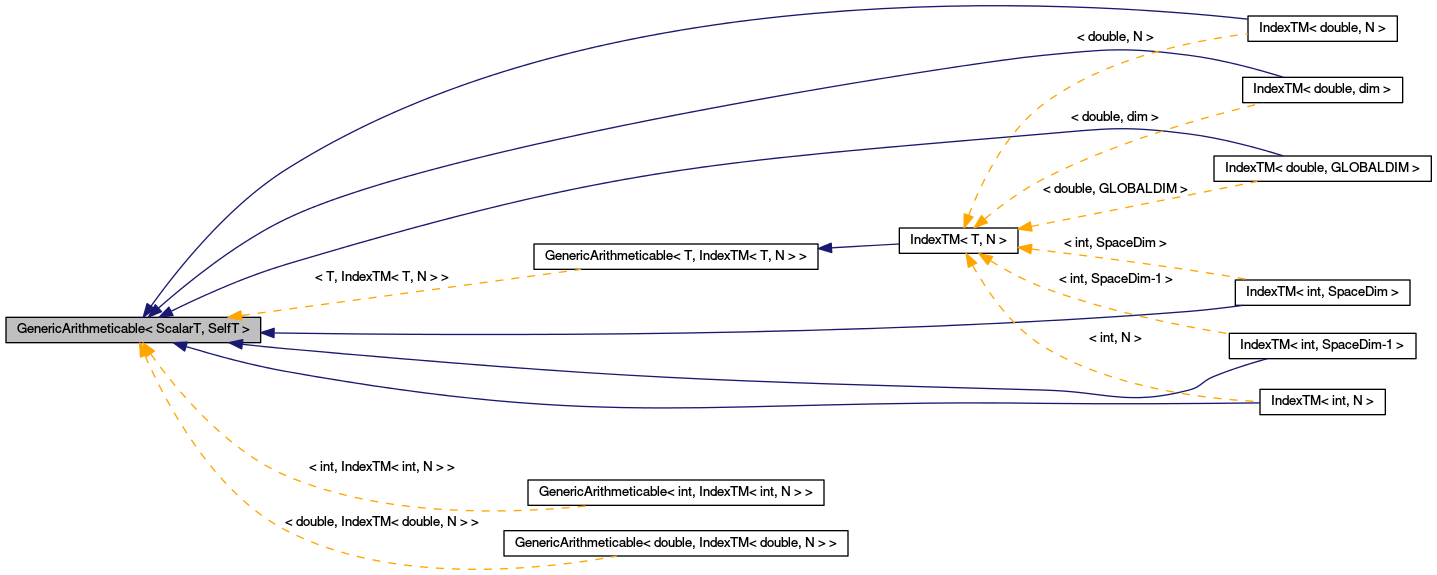# GenericArithmeticable< ScalarT, SelfT > Struct Template Reference

`#include <GenericArithmetic.H>`

Inheritance diagram for GenericArithmeticable< ScalarT, SelfT >:[legend]

## Detailed Description

### template<typename ScalarT, typename SelfT> struct GenericArithmeticable< ScalarT, SelfT >

Class that you need to derive from, if you want the *global* operators below to be available for your class. For example: class Foo : public GenericArithmeticable<double,Foo> { public: Foo() : GenericArithmeticable<double,Foo>(this) { } Foo& operator+=( const Foo& ); Foo& operator+=( const double& ); [etc] };

If, in addition, you want the member-function operators, you'll need to implement some generic operator-appliers in your derived class: see, in GenericArithmeticI.H, operatorCompare() and operatorOpEquals(). If you implement operator<, operator+= etc in your derived class, then that'll override the ones here (regardless of whether you've defined operatorCompare() and operatorOpEquals() in your derived class, though it would then be weird if you did).

## Public Types

typedef ScalarT scalar_type
typedef SelfT self_type

## Public Member Functions

virtual ~GenericArithmeticable ()
GenericArithmeticable (SelfT *s)
bool operator< (const SelfT &) const
bool operator> (const SelfT &) const
bool operator<= (const SelfT &) const
bool operator>= (const SelfT &) const
SelfT & operator+= (const SelfT &)
SelfT & operator+= (const ScalarT &)
SelfT & operator-= (const SelfT &)
SelfT & operator-= (const ScalarT &)
SelfT & operator*= (const SelfT &)
SelfT & operator*= (const ScalarT &)
SelfT & operator/= (const SelfT &)
SelfT & operator/= (const ScalarT &)

SelfT * m_child

## Member Typedef Documentation

template<typename ScalarT, typename SelfT>
 typedef ScalarT GenericArithmeticable< ScalarT, SelfT >::scalar_type

template<typename ScalarT, typename SelfT>
 typedef SelfT GenericArithmeticable< ScalarT, SelfT >::self_type

## Constructor & Destructor Documentation

template<typename ScalarT, typename SelfT>
 virtual GenericArithmeticable< ScalarT, SelfT >::~GenericArithmeticable ( ) ` [inline, virtual]`

template<typename ScalarT, typename SelfT>
 GenericArithmeticable< ScalarT, SelfT >::GenericArithmeticable ( SelfT * s ) ` [inline]`

## Member Function Documentation

template<typename ScalarT, typename SelfT>
 bool GenericArithmeticable< ScalarT, SelfT >::operator< ( const SelfT & rhs ) const` [inline]`

Reimplemented in IndexTM< T, N >.

template<typename ScalarT, typename SelfT>
 bool GenericArithmeticable< ScalarT, SelfT >::operator> ( const SelfT & rhs ) const` [inline]`

template<typename ScalarT, typename SelfT>
 bool GenericArithmeticable< ScalarT, SelfT >::operator<= ( const SelfT & rhs ) const` [inline]`

template<typename ScalarT, typename SelfT>
 bool GenericArithmeticable< ScalarT, SelfT >::operator>= ( const SelfT & rhs ) const` [inline]`

template<typename ScalarT, typename SelfT>
 SelfT & GenericArithmeticable< ScalarT, SelfT >::operator+= ( const SelfT & rhs ) ` [inline]`

template<typename ScalarT, typename SelfT>
 SelfT & GenericArithmeticable< ScalarT, SelfT >::operator+= ( const ScalarT & a ) ` [inline]`

template<typename ScalarT, typename SelfT>
 SelfT & GenericArithmeticable< ScalarT, SelfT >::operator-= ( const SelfT & rhs ) ` [inline]`

template<typename ScalarT, typename SelfT>
 SelfT & GenericArithmeticable< ScalarT, SelfT >::operator-= ( const ScalarT & a ) ` [inline]`

template<typename ScalarT, typename SelfT>
 SelfT & GenericArithmeticable< ScalarT, SelfT >::operator*= ( const SelfT & rhs ) ` [inline]`

template<typename ScalarT, typename SelfT>
 SelfT & GenericArithmeticable< ScalarT, SelfT >::operator*= ( const ScalarT & a ) ` [inline]`

template<typename ScalarT, typename SelfT>
 SelfT & GenericArithmeticable< ScalarT, SelfT >::operator/= ( const SelfT & rhs ) ` [inline]`

template<typename ScalarT, typename SelfT>
 SelfT & GenericArithmeticable< ScalarT, SelfT >::operator/= ( const ScalarT & a ) ` [inline]`

## Member Data Documentation

template<typename ScalarT, typename SelfT>
 SelfT* GenericArithmeticable< ScalarT, SelfT >::m_child` [private]`

The documentation for this struct was generated from the following files:

Generated on Mon Mar 25 04:25:04 2019 for Chombo + EB by1.5.5